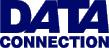The British Informatics Olympiad is the computing competition for schools and colleges. We are proud to present BIO'98.The 1998 British Informatics Olympiad exam

Question 1Question 1 Roman Numerals In Roman times, numbers were represented using letters. The way of doing this, known as Roman Numerals, is often seen depicting the copyright date on films and television.Roman numerals are conventionally defined to represent numbers using seven letters: I=1, V=5, X=10, L=50, C=100, D=500 and M=1000. Numbers other than these are formed by placing letters together from left to right, in descending order of size, and adding their values. The basic rule is to always use the biggest numeral possible (e.g. 15 is represented as XV, but never as VVV, VX or XIIIII). Letters may not appear more than three times in a row, so there are six exceptions to these rules - the combinations IV, IX, XL, XC, CD and CM. In these cases a letter is placed before one of greater value, and the smaller value is subtracted from the larger, e.g. CD = 400. Examples: ```26 XXVI 94 XCIV 555 DLV 1998 MCMXCVIII``` 1 (a) [20 marks] Write a program which accepts a number, between 1 and 3999 inclusive, and outputs the same number in Roman numerals. Sample run 1999 MCMXCIX
 1 (b) [4 marks] (i) What is LXIII + XXXVIII? Give your answer in Roman numerals. (ii) What is MCCXLIX + DCXV? Give your answer in Roman numerals. 1 (c) [6 marks] Some Roman numerals have fewer characters than the number of digits in the number they represent, e.g. C (1 character) compared with 100 (3 digits). Similarly some have more characters (e.g. XXIV and 24), and some have the same length (e.g. V and 5). How many of the numbers from 1 to 3999 inclusive have fewer characters in their Roman numeral form, and how many have more?